## Three capacitors having capacitances of 8.40, 8.40, and 4.20μF, respectively, are connected in series across a 36.0-V potential difference.

Question

Three capacitors having capacitances of 8.40, 8.40, and 4.20μF, respectively, are connected in series across a 36.0-V potential difference. (a) What is the charge on the 4.20−μF capacitor? (b) The capacitors are disconnected from the potential difference without allowing them to discharge. They are then reconnected in parallel with each other with the positively charged plates connected together. What is the voltage across each capacitor in the parallel combination?

in progress 0
2 weeks 2021-08-30T19:30:23+00:00 1 Answers 0 views 0

Therefore,

The charge on the 4.20μF capacitor is 75.6μC.

The voltage across each capacitor in the parallel combination is 10.8 Volt.

Explanation:

Given:

Three capacitors having capacitance,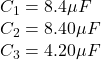are connected in series across a 36.0-V potential difference,

V= 36 V

To Find:

Qs = ? charge on the 4.20−μF

V = ? voltage across each capacitor in the parallel combination

Solution:

For Capacitor Series Combination we have,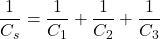Substituting the values we get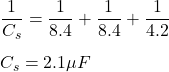As Capacitors are connected in series they have the same charge on each plates, and is given by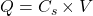Substituting the values we get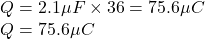Now when the plates are connected in Parallel we will have,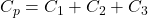Substituting the values we get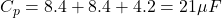As Capacitors are connected in Parallel they have the same Voltage on each plates, and is given by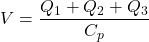Substituting the values we get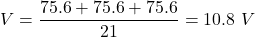Therefore,

The charge on the 4.20μF capacitor is 75.6μC.

The voltage across each capacitor in the parallel combination is 10.8 Volt.